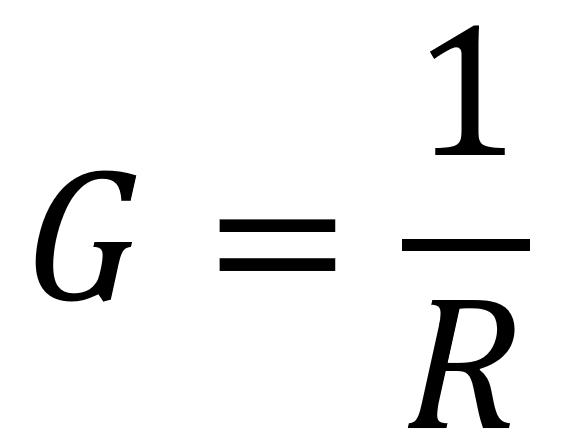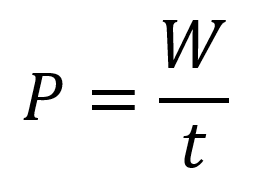# Formulas### Conductance Formula

Introduction Conductance    The reciprocal of resistance is conductance, symbolized by . It is a measurement of the ease, with which current is established. The formula is The unit of conductance...

### Conductance Formula

Introduction Conductance    The reciprocal of resistance is conductance, symbolized by . It is a measurement of the ease, with which current is established. The formula is The unit of conductance...### Energy & Power Formula

0.0 Introduction Energy is the ability to do work, and power is the rate at which energy is used. Power ( ) is a certain amount of energy ( )...

### Energy & Power Formula

0.0 Introduction Energy is the ability to do work, and power is the rate at which energy is used. Power ( ) is a certain amount of energy ( )...